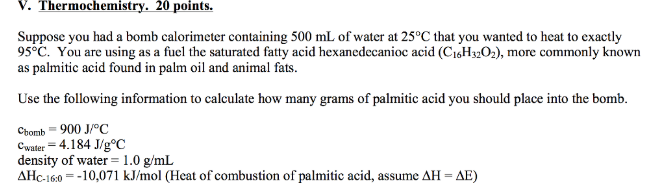# Problem: Suppose you had a bomb calorimeter containing 500 mL of water at 25°C that you wanted to heat to exactly 95°C. You are using as a fuel the saturated fatty acid hexanedecanioc acid (C16H32O2), more commonly known as palmitic acid found in palm oil and animal fats. Use the following information to calculate how many grams of palmitic acid you should place into the bomb.Cbomb = 900 J/°C Cwater = 4.184 J/g°C density of water = 1.0 g/mL ΔHC-16.0 = 10, 071 kJ/mol (Heat of combustion of palmitic acid, assume ΔH = ΔE)

###### FREE Expert Solution
87% (415 ratings)###### Problem Details

Suppose you had a bomb calorimeter containing 500 mL of water at 25°C that you wanted to heat to exactly 95°C. You are using as a fuel the saturated fatty acid hexanedecanioc acid (C16H32O2), more commonly known as palmitic acid found in palm oil and animal fats.

Use the following information to calculate how many grams of palmitic acid you should place into the bomb.
Cbomb = 900 J/°C

Cwater = 4.184 J/g°C

density of water = 1.0 g/mL

ΔHC-16.0 = 10, 071 kJ/mol (Heat of combustion of palmitic acid, assume ΔH = ΔE)